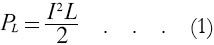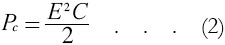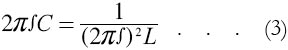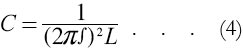# The Use of Electric Condensers or Capacitors

(Reproduced from "Zero to Eighty" pp.318-320 by EF Northrup)

When inductive devices such as the coils of an electric gun are used on alternating current, a certain portion of the power capacity of the supply system must be used to furnish reactive power. This reactive power is being stored up as electromagnetic energy in the coils or inductors at one moment, and at another moment the electromagnetic energy is being returned to the power source. This exchange of re-active power occurs twice per cycle, and hence prevents the power source from delivering its full quota of energy in the form of heat or motion.

It so happens that electric condensers or capacitors also take reactive power when connected to alternating current. In certain ways, this kind of reactive power is directly opposite in character to that taken by a coil or inductor connected to the same source; that is, the capacitor stores up electrostatic energy, whereas the inductor stores up electromagnetic energy.

Under certain conditions the leading reactive power of one or a group of capacitors can be so adjusted as to equal the lagging reactive power of one or a group of inductors, under which condition the power supply is relieved of all reactive component.

The reactive component of power (sometimes known as wattless current) thus alternately appears in the electrostatic and the electromagnetic forms and circulates between the inductive and condensive parts of the system. The expression for the energy stored up in the electromagnetic form is given as:where PL is the stored-up electromagnetic energy in joules, I the maximum value of the current in amperes, and L the inductance in henrys. The corresponding expression for the energy stored up in the electro-static form is:where Pc is the stored-up electrostatic energy in joules, E the maximum value of the potential in volts, and C the capacity in farads.

When the source of electric power produces an alternating current which varies in a substantially sinusoidal manner, it can be shown that the numerical relation of C and L can be more simply derived by the use of the formula:where C and L have the same meaning as above, the constant pi its usual mathematical significance, and f is the frequency in cycles of the alternating current supply.

In many cases, the value of f, the frequency, is given; and L can be measured or calculated. Knowing f and L we may write:by which we can calculate the exact value of electrostatic capacity necessary to counterbalance the inductance in the system. In practice, it is not always possible to connect the numerical values of C and L by the simple formula (4). However, where various complexities due to coupled circuits or voltage transformations arise, the more fundamental equations (1) and (2) must be equated at all times; otherwise the power source must carry more or less reactive component.

When the equivalent inductive and condensive reactances of a sinusoidal a.c. circuit are equal, the circuit is said to be resonated. When resonated, some circuits have many times the power (expressed as reactive K-VA) of the source of the alternating current. This property of resonated circuits is especially valuable in the case of the electric gun. By using this principle, a great deal of electromagnetic energy can be secured without the necessity of having a correspond-ing amount of generator capacity. For frequencies a thousand cycles or above, standard capacitor units are made which, although small, will deliver many times the reactive power that could be produced by any generator of the same size and cost.

 < Previous Page 9 of 17 Next >